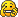## "If" Scripts

### "If" Scripts

Could some post an example of "if" in thyme
Say something like: If "controlleracc" is "<1" then e.object.density=10
Newbie Thymer
Intermediate Phuner
Cs24

Posts: 41
Joined: Tue Nov 03, 2009 5:30 am

### Re: "If" Scripts

viewtopic.php?f=13&t=411
It's right there.
Millions of voices suddenly cried out in terror, and were suddenly silenced. Something terrible has happened.RicH
[Funniest Person 2010]

Posts: 2043
Joined: Tue Sep 01, 2009 9:01 am

### Re: "If" Scripts

(e)=>{e.geom.controlleracc == <0 ? {e.geom.density = 0}}

This won't work nor does "<="
but it will [work] if I remove the <
Fixes?
Newbie Thymer
Intermediate Phuner
Cs24

Posts: 41
Joined: Tue Nov 03, 2009 5:30 am

### Re: "If" Scripts

Cs24 wrote:
(e)=>{e.geom.controlleracc == <0 ? {e.geom.density = 0}}

This won't work nor does "<="
but it will [work] if I remove the <
Fixes?

If structures take a boolean value (true or false) and if it's true, run the first script and if false, run the second script, you always need three inputs for an if structure, for example:
Code: Select all
`e.geom.controlleracc == 0 ? {print("hello")} : {};`

should print "hello" in the console (view by pressing F11 or Tilde("~")) if e.geom.controlleracc is equal to 0.

Different statements you can check are:
x == y, x is equal to y.
x != y, x is not equal to y.
x < y, x is less than y.
x > y, x is more than y.
x <= y, x is less than or equal to y.
x >= y, x is more than or equal to y.

Each of these statements return a true or false value, depending on whether they are true or false.

Another thing you can check for is OR (represented as ||), if either inputs or both inputs are true, it will return true, otherwise it will return false, for example true || false will return true, or x == y || x != y will return true, or, false || false will return false similarly, false || true will return true as well.

You can also check for AND (represented as &&), if both inputs are true, it will return true, if either input or both inputs are false, it will return false, for example true && true will return true, whereas false && true or false && false will return false.

These can be combined a lot to form the questions you want, like for example:
Code: Select all
`(Value1 != Value2 && Value2 == Value3) || (Value3 == Value1 && Value2 == Value4) ? {print("Success")} : {print("Try again")};`

The code I think you want is:
Code: Select all
`(e)=>{e.geom.controlleracc < 0 ? {e.geom.density = 0} : {}}`

Hopefully this has answered your question, if not, please be very specific.
Mechanisms: 18 Mechanisms.
Thyme: Tutorial - Variables/Commands List.
Thymechanic

Posts: 174
Joined: Tue Sep 01, 2009 8:33 am
Location: Victoria, Australia

### Re: "If" Scripts

gradyfitz wrote:Different statements you can check are:
x == y, x is equal to y.
x != y, x is not equal to y.
x < y, x is less than y.
x > y, x is more than y.
x <= y, x is less than or equal to y.
x >= y, x is more than or equal to y.

Thanks!Newbie Thymer
Intermediate Phuner
Cs24

Posts: 41
Joined: Tue Nov 03, 2009 5:30 am

### Re: "If" Scripts

also, there is another way to create if-statements, the if_then_else(condition, what to do if true, what to do if false) function.
Jrv wrote:
TC42 wrote:Quite honestly, I didn't think anyone on 4chan has that good a use of grammar, spelling, usage, mechanics, ect.
But I've never been there, so I may be wrong.

GTFO newfgtRA2lover

Posts: 607
Joined: Mon Aug 31, 2009 8:43 pm
Location: Brazil